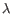## The statistical model

Each gene is modeled by a separate Generalized Linear Model (GLM). The use of the GLM formalism allows us to fit curves to expression values without assuming that the error on the values is normally distributed. Similarly to EdgeR and DESeq, we assume that the read counts follow a Negative Binomial distribution.

The Negative Binomial distribution can be understood as a `Gamma-Poisson' mixture distribution i.e. the distribution resulting from a mixture of Poisson distributions, where the Poisson parameteris itself Gamma-distributed. In an RNA-Seq context, this Gamma distribution is controlled by the dispersion parameter, such that the Negative Binomial distribution reduces to a Poisson distribution when the dispersion is zero.

Subsections